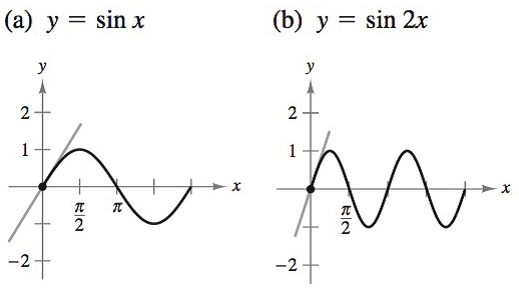Chapter 8.4, Problem 41E### Calculus: An Applied Approach (Min...

10th Edition
Ron Larson
ISBN: 9781305860919

#### Solutions

Chapter
Section### Calculus: An Applied Approach (Min...

10th Edition
Ron Larson
ISBN: 9781305860919
Textbook Problem
1 views

# Slope of a Tangent Line In Exercises 41 and 42, find the slope of the tangent line to the given sine function at the origin. What can you conclude about the slope of the sine function sin ax at the origin? (a)  y = sin x                   (b)  y = sin 2 x(a)

To determine

To calculate: The slope of the tangent line to the graph of the provided sine function y=sinx at the origin and provided its graph, which is shown below as:Explanation

Given Information:

The provided function is y=sinx and its graph is shown below as:

Formula used:

Sine differentiation rule:

ddx[sinu]=cosududx

General power rule of differentiation:

ddx[xn]=nxn1

Calculation:

Consider the provided function:

y=sinx

Differentiate the above function using Sine and general power rules of differentiation

(b)

To determine

To calculate: The slope of the tangent line to the graph of the provided sine function y=sin2x at the origin and provided its graph, which is shown below as:### Still sussing out bartleby?

Check out a sample textbook solution.

See a sample solution

#### The Solution to Your Study Problems

Bartleby provides explanations to thousands of textbook problems written by our experts, many with advanced degrees!

Get Started

#### In Exercises 3540, rationalize the numerator of each expression. 36. y3x

Applied Calculus for the Managerial, Life, and Social Sciences: A Brief Approach

#### In problems 1-16, solve each equation. 16.. Solve

Mathematical Applications for the Management, Life, and Social Sciences

#### True or False: converges.

Study Guide for Stewart's Single Variable Calculus: Early Transcendentals, 8th

#### Define a construct and explain the role that constructs play in theories.

Research Methods for the Behavioral Sciences (MindTap Course List)